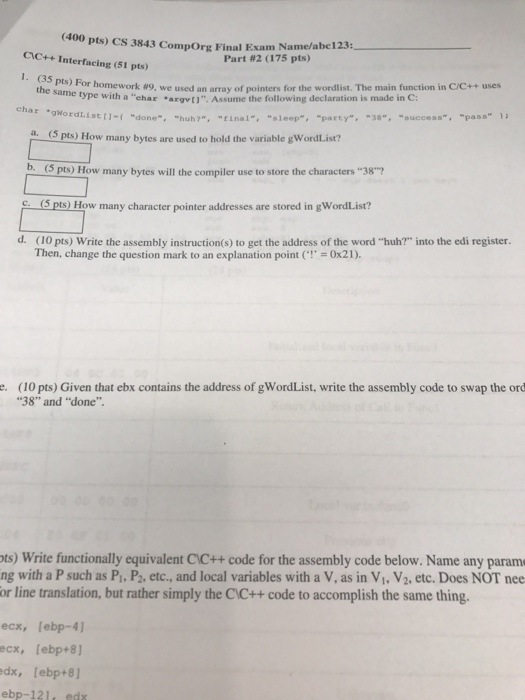# Question & Answer: main function in C/C++ uses the same type with a "char argv[]". Assume the foll…..Help no 1

For homework #9, we used an array of pointers for the wordlist. The main function in C/C++ uses the same type with a “char argv[]”. Assume the following declaration is made in C: char gWordList [] = { “done”, “huh?”, “final”, “sleep”, “party”, “38”, “success”, “pass” }: a. How many bytes are used to hold the variable gWordList? b. How many bytes will the compiler use to store the characters “38”? c. How many character pointer addresses are stored in gWordList? d. Write the assembly instruction(s) to get the address of the word “huh?” into the edi register. Then, change the question mark to an explanation point (‘!’ = 0 times 21). e. Given that ebx contains the address of gWordList, write the assembly code to swap the ord “38” and “done”. Write functionally equivalent CC++ code for the assembly code below. Name any parame with a P such as P_1, P_2, etc., and local variables with a V, as in V_1, V_2, etc. Does NOT need line translation, but rather simply the CC++ code to accomplish the same thing.

1.

Don't use plagiarized sources. Get Your Custom Essay on
Question & Answer: main function in C/C++ uses the same type with a "char argv[]". Assume the foll…..
GET AN ESSAY WRITTEN FOR YOU FROM AS LOW AS \$13/PAGE

a.

gWordList is an array of pointers and a pointer will occupy 4 bytes, here we have

a total of 8 elements in the array hence it will take 8*4 = 32 bytes of space.

b.

A character will occupy 1 byte hence for “38” complier will take 2 bytes to store.

c.

In the list there are 8 elements hence 8 char pointers addresses are stored.

d.

mov edi, gWordList

mov eax, [edi+4]       ; this will read second element “huh?”

mov byte ptr [eax+3], 21h   ; this will replace ? with !